## NCERT Solutions for Class 9 Maths Chapter 13 Surface Areas and Volumes Ex 13.4

NCERT Solutions for Class 9 Maths Chapter 13 Surface Areas and Volumes Ex 13.4 are part of NCERT Solutions for Class 9 Maths. Here we have given NCERT Solutions for Class 9 Maths Chapter 13 Surface Areas and Volumes Ex 13.4.

 Board CBSE Textbook NCERT Class Class 9 Subject Maths Chapter Chapter 13 Chapter Name Surface Areas and Volumes Exercise Ex 13.4 Number of Questions Solved 9 Category NCERT Solutions

## NCERT Solutions for Class 9 Maths Chapter 13 Surface Areas and Volumes Ex 13.4

Question 1.
Find the surface area of a sphere of radius
(i) 10.5 cm
(ii) 5.6 cm
(iii) 14 cm
Solution:
(i) We have, r = 105 cm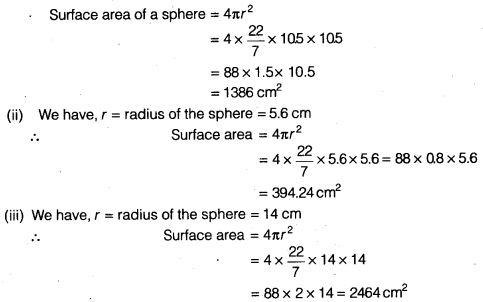Question 2.
Find the surface area of a sphere of diameter
(i) 14 cm
(ii) 21 cm
(iii) 3.5 m
Solution: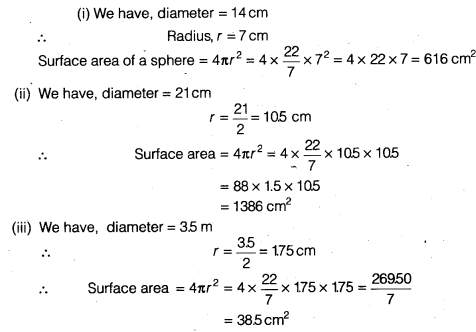Question 3.
Find the total surface area of a hemisphere of radius 10 cm. (Use π = 3.14)
Solution:
We have, r = 10 cm
Total surface area of a hemisphere = 3πr2
= 3 x 3.14 x (10)2
= 9.42 x 100
= 942 cm2

Question 4.
The radius of a spherical balloon increases from 7 cm to 14 cm as air is being pumped into it. Find the ratio of surface areas of the balloon in the two cases.
Solution:
Let initial radius, r1 = 7 cm
After increases, r2 = 14 cm
Surface area for initial balloon = 4πr12 = 4 x $$\frac { 22 }{ 7 }$$ x 7 x 7 = 88 x 7
A1 = 616 cm2
Surface area for increasing balloon = 4πr22 = 4x $$\frac { 22 }{ 7 }$$ x 14 x 14 = 88 x 28
A2 = 2464 cm2
∴ Required ratio = A1 : A2 = 616 : 2464 = 1 : 4

Question 5.
A hemispherical bowl made of brass has inner diameter 10.5 cm. Find the cost of tin-plating it on the inside at the rate of ₹16 per 100 cm2.
Solution:
We have, inner diameter = 10.5 cm
Inner radius = $$\frac { 10.5 }{ 2 }$$ cm = 5.25 cm
Curved surface area of hemispherical bowl of inner side = 2πr2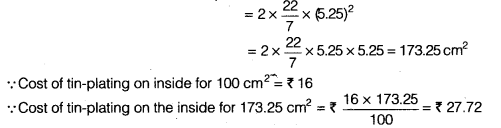Question 6.
Find the radius of a sphere whose surface area is 154 cm2.
Solution:
Surface area of a sphere = 154 cm2Hence, the radius of the sphere is 3.5 cm.

Question 7.
The diameter of the Moon is approximately one-fourth of the diameter of the Earth. Find the ratio of their surface areas.
Solution:
Let diameter of the Earth = d1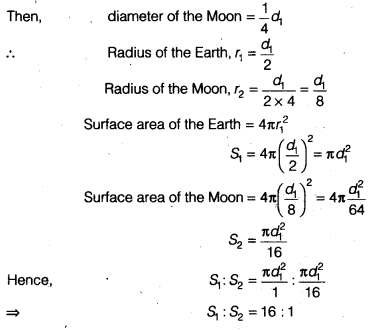Question 8.
A hemispherical bowl is made of steel, 0.25 cm thick. The inner radius of the bowl is 5 cm. Find the outer curved surface area of the bowl.
Solution:
= ( 5 + 0.25) cm = 5.25 cm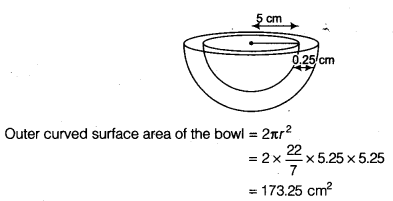Question 9.
A right circular cylinder just encloses a sphere of radius r (see figure). Find
(i) surface area of the sphere,
(ii) curved surface area of the cylinder,
(iii) ratio of the areas obtained in (i) and (ii).
Solution: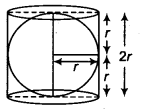The radius of the sphere = r